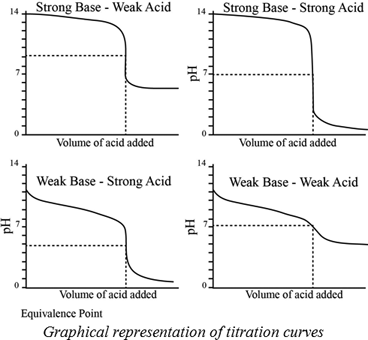# Using Figure 17.11, suggest an indicator to use in each of the following titrations. (a) NaHCO 3 is titrated to CO 3 2− with NaOH. (b) Hypochlorous acid is titrated with NaOH. (c) Trimethylamine is titrated with HCl.### Chemistry & Chemical Reactivity

9th Edition
John C. Kotz + 3 others
Publisher: Cengage Learning
ISBN: 9781133949640

#### Solutions

Chapter
Section### Chemistry & Chemical Reactivity

9th Edition
John C. Kotz + 3 others
Publisher: Cengage Learning
ISBN: 9781133949640
Chapter 17, Problem 36PS
Textbook Problem
359 views

## Using Figure 17.11, suggest an indicator to use in each of the following titrations. (a) NaHCO3 is titrated to CO32− with NaOH. (b) Hypochlorous acid is titrated with NaOH. (c) Trimethylamine is titrated with HCl.

a)

Interpretation Introduction

Interpretation:

NaHCO3 is titrated to CO32- with NaOH; an indicator used has to be suggested.

Concept introduction:

A titration is one of the most useful ways of determining accurately the quantity of an acid, a base or some other substances in a mixture.

The pH at the equivalence point of a strong acid –strong base titration is 7.

A weak acid titrated with a strong base leads to a pH > 7 at the equivalence point.

A weak base titrated with a strong acid leads to pH < 7 at the equivalence point.Indicators:

A chemical substances which gives a visible change  in the titration.

Some important indicators are as follow.### Explanation of Solution

HCO3- is a weak acid and pKa= 10.32. When a weak acid is titrated with a strong base the pH at the equivalence point is above 7.

HCO3- have high pKa value therefore, it is weak base

b)

Interpretation Introduction

Interpretation:

Hypochlorous acid titrated with NaOH; an indicator used has to be suggested.

Concept introduction:

A titration is one of the most useful ways of determining accurately the quantity of an acid, a base or some other substances in a mixture.

The pH at the equivalence point of a strong acid –strong base titration is 7.

A weak acid titrated with a strong base leads to a pH > 7 at the equivalence point.

A weak base titrated with a strong acid leads to pH < 7 at the equivalence point.Indicators:

A chemical substances which gives a visible change  in the titration.

Some important indicators are as follow.c)

Interpretation Introduction

Interpretation:

Trimethylamine is titrated with HCl ; an indicator used has to be suggested.

Concept introduction:

A titration is one of the most useful ways of determining accurately the quantity of an acid, a base or some other substances in a mixture.

The pH at the equivalence point of a strong acid –strong base titration is 7.

A weak acid titrated with a strong base leads to a pH > 7 at the equivalence point.

A weak base titrated with a strong acid leads to pH < 7 at the equivalence point.Indicators:

A chemical substances which gives a visible change  in the titration.

Some important indicators are as follow.### Still sussing out bartleby?

Check out a sample textbook solution.

See a sample solution

#### The Solution to Your Study Problems

Bartleby provides explanations to thousands of textbook problems written by our experts, many with advanced degrees!

Get Started

Find more solutions based on key concepts
Fried banana or vegetable snack chips make a healthy everyday snack choice for vegetarians. T F

Nutrition: Concepts and Controversies - Standalone book (MindTap Course List)

An electric drill with a steel drill bit of mass m = 27.0 g and diameter 0.635 cm is used to drill into a cubic...

Physics for Scientists and Engineers, Technology Update (No access codes included)

Think for a moment: What does the term local group suggest?

Oceanography: An Invitation To Marine Science, Loose-leaf Versin